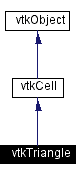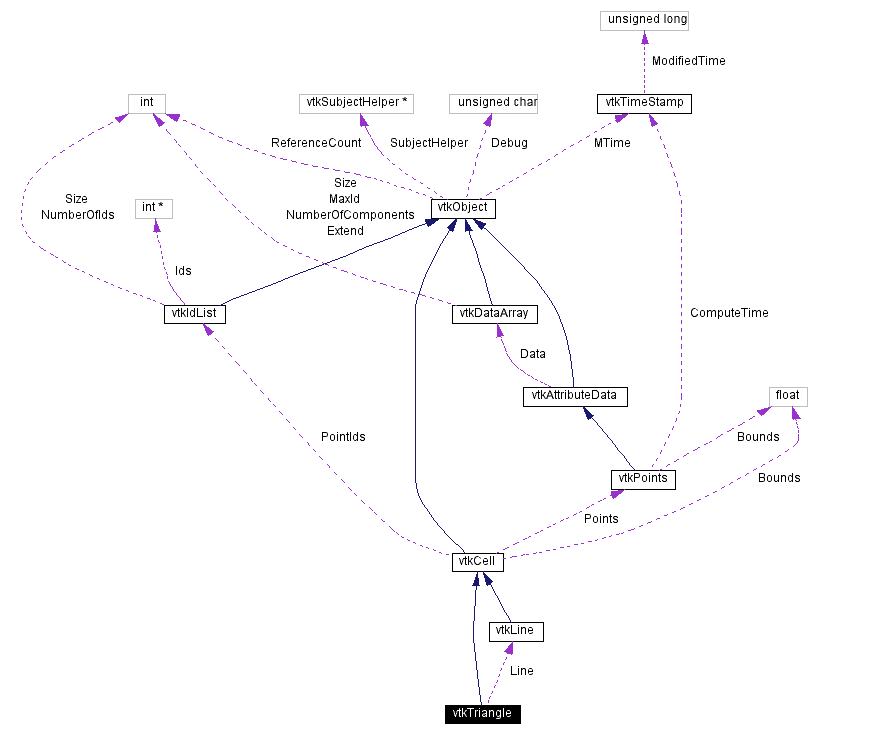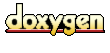Main Page   Class Hierarchy   Alphabetical List   Compound List   File List   Compound Members   File Members   Related Pages

# vtkTriangle Class Reference

a cell that represents a triangle. More...

`#include <vtkTriangle.h>`

Inheritance diagram for vtkTriangle:[legend]
Collaboration diagram for vtkTriangle:[legend]
List of all members.

## Public Methods

virtual const char * GetClassName ()
virtual int IsA (const char *type)
vtkCellMakeObject ()
vtkCellGetEdge (int edgeId)
int GetCellType ()
int GetCellDimension ()
int GetNumberOfEdges ()
int GetNumberOfFaces ()
vtkCellGetFace (int)
int CellBoundary (int subId, float pcoords, vtkIdList *pts)
void Contour (float value, vtkScalars *cellScalars, vtkPointLocator *locator, vtkCellArray *verts, vtkCellArray *lines, vtkCellArray *polys, vtkPointData *inPd, vtkPointData *outPd, vtkCellData *inCd, int cellId, vtkCellData *outCd)
int EvaluatePosition (float x, float *closestPoint, int &subId, float pcoords, float &dist2, float *weights)
void EvaluateLocation (int &subId, float pcoords, float x, float *weights)
int Triangulate (int index, vtkIdList *ptIds, vtkPoints *pts)
void Derivatives (int subId, float pcoords, float *values, int dim, float *derivs)
void Clip (float value, vtkScalars *cellScalars, vtkPointLocator *locator, vtkCellArray *polys, vtkPointData *inPd, vtkPointData *outPd, vtkCellData *inCd, int cellId, vtkCellData *outCd, int insideOut)
int IntersectWithLine (float p1, float p2, float tol, float &t, float x, float pcoords, int &subId)
int GetParametricCenter (float pcoords)
int CellBoundary (int subId, float pcoords, vtkIdList &pts)
int Triangulate (int index, vtkIdList &ptIds, vtkPoints &pts)

## Static Public Methods

vtkTriangle * New ()
int IsTypeOf (const char *type)
vtkTriangle * SafeDownCast (vtkObject *o)
void TriangleCenter (float p1, float p2, float p3, float center)
float TriangleArea (float p1, float p2, float p3)
double Circumcircle (double p1, double p2, double p3, double center)
int BarycentricCoords (double x, double x1, double x2, double x3, double bcoords)
int ProjectTo2D (double x1, double x2, double x3, double v1, double v2, double v3)
void ComputeNormal (vtkPoints *p, int numPts, int *pts, float n)
void ComputeNormal (float v1, float v2, float v3, float n)
void ComputeNormalDirection (float v1, float v2, float v3, float n)
void ComputeNormal (double v1, double v2, double v3, double n)
void ComputeNormalDirection (double v1, double v2, double v3, double n)
int PointInTriangle (float x, float x1, float x2, float x3, float tol2)

## Protected Methods

vtkTriangle ()
~vtkTriangle ()
vtkTriangle (const vtkTriangle &)
void operator= (const vtkTriangle &)

vtkLineLine

## Detailed Description

a cell that represents a triangle.

Date:
2000/12/10 20:08:20
Revision:
1.63

vtkTriangle is a concrete implementation of vtkCell to represent a triangle located in 3-space.

Examples:
vtkTriangle (examples)

Definition at line 61 of file vtkTriangle.h.

## Constructor & Destructor Documentation

 vtkTriangle::vtkTriangle ( ) ` [protected]`

 vtkTriangle::~vtkTriangle ( ) ` [protected]`

 vtkTriangle::vtkTriangle ( const vtkTriangle & ) ` [inline, protected]`
 Definition at line 196 of file vtkTriangle.h.

## Member Function Documentation

 vtkTriangle* vtkTriangle::New ( ) ` [static]`
 Create an object with Debug turned off, modified time initialized to zero, and reference counting on. Reimplemented from vtkObject.

 virtual const char* vtkTriangle::GetClassName ( ) ` [virtual]`
 Return the class name as a string. This method is defined in all subclasses of vtkObject with the vtkTypeMacro found in vtkSetGet.h. Reimplemented from vtkCell.

 int vtkTriangle::IsTypeOf ( const char * type ) ` [static]`
 Return 1 if this class type is the same type of (or a subclass of) the named class. Returns 0 otherwise. This method works in combination with vtkTypeMacro found in vtkSetGet.h. Reimplemented from vtkCell.

 virtual int vtkTriangle::IsA ( const char * type ) ` [virtual]`
 Return 1 if this class is the same type of (or a subclass of) the named class. Returns 0 otherwise. This method works in combination with vtkTypeMacro found in vtkSetGet.h. Reimplemented from vtkCell.

 vtkTriangle* vtkTriangle::SafeDownCast ( vtkObject * o ) ` [static]`
 Will cast the supplied object to vtkObject* is this is a safe operation (i.e., a safe downcast); otherwise NULL is returned. This method is defined in all subclasses of vtkObject with the vtkTypeMacro found in vtkSetGet.h. Reimplemented from vtkCell.

 vtkCell* vtkTriangle::MakeObject ( ) ` [virtual]`
 Create a new cell and copy this triangle's information into the cell. Returns a pointer to the new cell created. Reimplemented from vtkCell.

 vtkCell* vtkTriangle::GetEdge ( int edgeId ) ` [virtual]`
 Get the edge specified by edgeId (range 0 to 2) and return that edge's coordinates. Reimplemented from vtkCell.

 int vtkTriangle::GetCellType ( ) ` [inline, virtual]`
 See the vtkCell API for descriptions of these methods. Reimplemented from vtkCell. Definition at line 76 of file vtkTriangle.h.

 int vtkTriangle::GetCellDimension ( ) ` [inline, virtual]`
 Return the topological dimensional of the cell (0,1,2, or 3). Reimplemented from vtkCell. Definition at line 77 of file vtkTriangle.h.

 int vtkTriangle::GetNumberOfEdges ( ) ` [inline, virtual]`
 Return the number of edges in the cell. Reimplemented from vtkCell. Definition at line 78 of file vtkTriangle.h.

 int vtkTriangle::GetNumberOfFaces ( ) ` [inline, virtual]`
 Return the number of faces in the cell. Reimplemented from vtkCell. Definition at line 79 of file vtkTriangle.h.

 vtkCell* vtkTriangle::GetFace ( int faceId ) ` [inline, virtual]`
 Return the face cell from the faceId of the cell. Reimplemented from vtkCell. Definition at line 80 of file vtkTriangle.h.

 int vtkTriangle::CellBoundary ( int subId, float pcoords, vtkIdList * pts ) ` [virtual]`
 Given parametric coordinates of a point, return the closest cell boundary, and whether the point is inside or outside of the cell. The cell boundary is defined by a list of points (pts) that specify a face (3D cell), edge (2D cell), or vertex (1D cell). If the return value of the method is != 0, then the point is inside the cell. Reimplemented from vtkCell.

 void vtkTriangle::Contour ( float value, vtkScalars * cellScalars, vtkPointLocator * locator, vtkCellArray * verts, vtkCellArray * lines, vtkCellArray * polys, vtkPointData * inPd, vtkPointData * outPd, vtkCellData * inCd, int cellId, vtkCellData * outCd ) ` [virtual]`
 Generate contouring primitives. The scalar list cellScalars are scalar values at each cell point. The point locator is essentially a points list that merges points as they are inserted (i.e., prevents duplicates). Contouring primitives can be vertices, lines, or polygons. It is possible to interpolate point data along the edge by providing input and output point data - if outPd is NULL, then no interpolation is performed. Also, if the output cell data is non-NULL, the cell data from the contoured cell is passed to the generated contouring primitives. (Note: the CopyAllocate() method must be invoked on both the output cell and point data. The cellId refers to the cell from which the cell data is copied.) Reimplemented from vtkCell.

 int vtkTriangle::EvaluatePosition ( float x, float * closestPoint, int & subId, float pcoords, float & dist2, float * weights ) ` [virtual]`
 Given a point x return inside(=1) or outside(=0) cell; evaluate parametric coordinates, sub-cell id (!=0 only if cell is composite), distance squared of point x to cell (in particular, the sub-cell indicated), closest point on cell to x (unless closestPoint is null, in which case, the closest point and dist2 are not found), and interpolation weights in cell. (The number of weights is equal to the number of points defining the cell). Note: on rare occasions a -1 is returned from the method. This means that numerical error has occurred and all data returned from this method should be ignored. Also, inside/outside is determine parametrically. That is, a point is inside if it satisfies parametric limits. This can cause problems for cells of topological dimension 2 or less, since a point in 3D can project onto the cell within parametric limits but be "far" from the cell. Thus the value dist2 may be checked to determine true in/out. Reimplemented from vtkCell.

 void vtkTriangle::EvaluateLocation ( int & subId, float pcoords, float x, float * weights ) ` [virtual]`
 Determine global coordinate (x) from subId and parametric coordinates. Also returns interpolation weights. (The number of weights is equal to the number of points in the cell.) Reimplemented from vtkCell.

 int vtkTriangle::Triangulate ( int index, vtkIdList * ptIds, vtkPoints * pts ) ` [virtual]`
 Generate simplices of proper dimension. If cell is 3D, tetrahedron are generated; if 2D triangles; if 1D lines; if 0D points. The form of the output is a sequence of points, each n+1 points (where n is topological cell dimension) defining a simplex. The index is a parameter that controls which triangulation to use (if more than one is possible). If numerical degeneracy encountered, 0 is returned, otherwise 1 is returned. Reimplemented from vtkCell.

 void vtkTriangle::Derivatives ( int subId, float pcoords, float * values, int dim, float * derivs ) ` [virtual]`
 Compute derivatives given cell subId and parametric coordinates. The values array is a series of data value(s) at the cell points. There is a one-to-one correspondence between cell point and data value(s). Dim is the number of data values per cell point. Derivs are derivatives in the x-y-z coordinate directions for each data value. Thus, if computing derivatives for a scalar function in a hexahedron, dim=1, 8 values are supplied, and 3 deriv values are returned (i.e., derivatives in x-y-z directions). On the other hand, if computing derivatives of velocity (vx,vy,vz) dim=3, 24 values are supplied ((vx,vy,vz)1, (vx,vy,vz)2, ....()8), and 9 deriv values are returned ((d(vx)/dx),(d(vx)/dy),(d(vx)/dz), (d(vy)/dx),(d(vy)/dy), (d(vy)/dz), (d(vz)/dx),(d(vz)/dy),(d(vz)/dz)). Reimplemented from vtkCell.

 void vtkTriangle::Clip ( float value, vtkScalars * cellScalars, vtkPointLocator * locator, vtkCellArray * polys, vtkPointData * inPd, vtkPointData * outPd, vtkCellData * inCd, int cellId, vtkCellData * outCd, int insideOut ) ` [virtual]`
 Clip this triangle using scalar value provided. Like contouring, except that it cuts the triangle to produce other triangles. Reimplemented from vtkCell.

 int vtkTriangle::IntersectWithLine ( float p1, float p2, float tol, float & t, float x, float pcoords, int & subId ) ` [virtual]`
 Plane intersection plus in/out test on triangle. The in/out test is performed using tol as the tolerance. Reimplemented from vtkCell.

 int vtkTriangle::GetParametricCenter ( float pcoords ) ` [inline, virtual]`
 Return the center of the triangle in parametric coordinates. Reimplemented from vtkCell. Definition at line 203 of file vtkTriangle.h.

 void vtkTriangle::TriangleCenter ( float p1, float p2, float p3, float center ) ` [inline, static]`
 Compute the center of the triangle. Definition at line 267 of file vtkTriangle.h.

 float vtkTriangle::TriangleArea ( float p1, float p2, float p3 ) ` [inline, static]`
 Compute the area of a triangle in 3D. Definition at line 275 of file vtkTriangle.h.

 double vtkTriangle::Circumcircle ( double p1, double p2, double p3, double center ) ` [static]`
 Compute the circumcenter (center) and radius (method return value) of a triangle defined by the three points x1, x2, and x3. (Note that the coordinates are 2D. 3D points can be used but the z-component will be ignored.)

 int vtkTriangle::BarycentricCoords ( double x, double x1, double x2, double x3, double bcoords ) ` [static]`
 Given a 2D point x, determine the barycentric coordinates of the point. Barycentric coordinates are a natural coordinate system for simplices that express a position as a linear combination of the vertices. For a triangle, there are three barycentric coordinates (because there are three vertices), and the sum of the coordinates must equal 1. If a point x is inside a simplex, then all three coordinates will be strictly positive. If two coordinates are zero (so the third =1), then the point x is on a vertex. If one coordinates are zero, the point x is on an edge. In this method, you must specify the vertex coordinates x1->x3. Returns 0 if triangle is degenerate.

 int vtkTriangle::ProjectTo2D ( double x1, double x2, double x3, double v1, double v2, double v3 ) ` [static]`
 Project triangle defined in 3D to 2D coordinates. Returns 0 if degenerate triangle; non-zero value otherwise. Input points are x1->x3; output 2D points are v1->v3.

 void vtkTriangle::ComputeNormal ( vtkPoints * p, int numPts, int * pts, float n ) ` [static]`
 Compute the triangle normal from a points list, and a list of point ids that index into the points list.

 void vtkTriangle::ComputeNormal ( float v1, float v2, float v3, float n ) ` [inline, static]`
 Compute the triangle normal from three points. Definition at line 223 of file vtkTriangle.h.

 void vtkTriangle::ComputeNormalDirection ( float v1, float v2, float v3, float n ) ` [inline, static]`
 Compute the (unnormalized) triangle normal direction from three points. Definition at line 209 of file vtkTriangle.h. Referenced by ComputeNormal().

 void vtkTriangle::ComputeNormal ( double v1, double v2, double v3, double n ) ` [inline, static]`
 Compute the triangle normal from three points (double-precision version). Definition at line 252 of file vtkTriangle.h.

 void vtkTriangle::ComputeNormalDirection ( double v1, double v2, double v3, double n ) ` [inline, static]`
 Compute the (unnormalized) triangle normal direction from three points (double precision version). Definition at line 238 of file vtkTriangle.h.

 int vtkTriangle::PointInTriangle ( float x, float x1, float x2, float x3, float tol2 ) ` [static]`
 Given a point x, determine whether it is inside (within the tolerance squared, tol2) the triangle defined by the three coordinate values p1, p2, p3. Method is via comparing dot products. (Note: in current implementation the tolerance only works in the neighborhood of the three vertices of the triangle.

 void vtkTriangle::ComputeQuadric ( float x1, float x2, float x3, float quadric ) ` [static]`
 Calculate the error quadric for this triangle. Return the quadric as a 4x4 matrix or a vtkQuadric. (from Peter Lindstrom's Siggraph 2000 paper, "Out-of-Core Simplification of Large Polygonal Models")

 void vtkTriangle::ComputeQuadric ( float x1, float x2, float x3, vtkQuadric * quadric ) ` [static]`

 int vtkTriangle::CellBoundary ( int subId, float pcoords, vtkIdList & pts ) ` [inline]`
 For legacy compatibility. Do not use. Definition at line 186 of file vtkTriangle.h.

 int vtkTriangle::Triangulate ( int index, vtkIdList & ptIds, vtkPoints & pts ) ` [inline]`
 Definition at line 188 of file vtkTriangle.h.

 void vtkTriangle::operator= ( const vtkTriangle & ) ` [inline, protected]`
 Definition at line 197 of file vtkTriangle.h.

## Member Data Documentation

 vtkLine* vtkTriangle::Line` [protected]`
 Definition at line 199 of file vtkTriangle.h.

The documentation for this class was generated from the following file:

Generated on Wed Nov 21 13:01:20 2001 for VTK by1.2.11.1 written by Dimitri van Heesch, © 1997-2001